Скачать презентацию Unified Models of Dark Energy and Dark

d41549de0e4fb9fee69fe2993015515d.ppt

• Количество слайдов: 30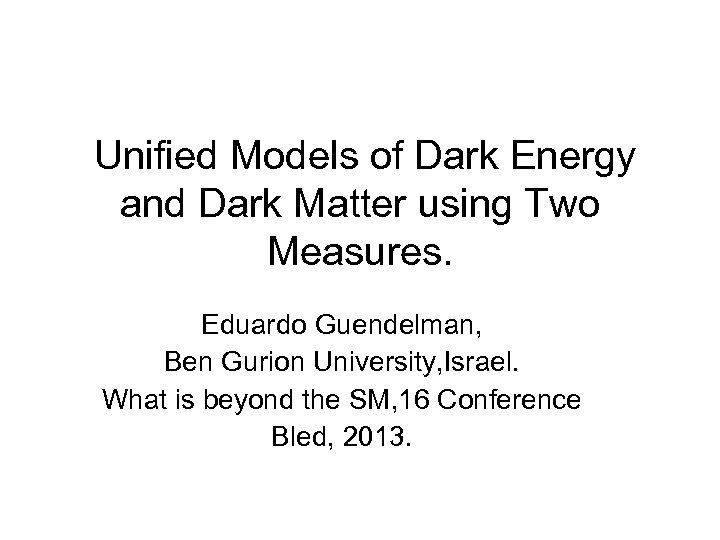Unified Models of Dark Energy and Dark Matter using Two Measures. Eduardo Guendelman, Ben Gurion University, Israel. What is beyond the SM, 16 Conference Bled, 2013.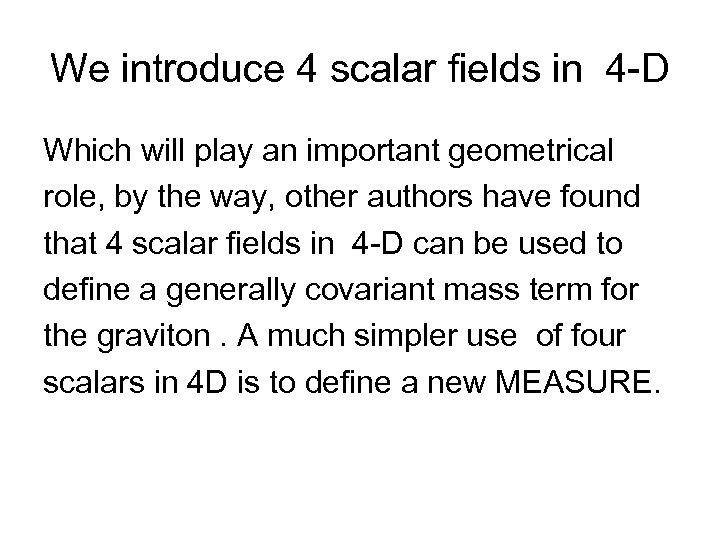We introduce 4 scalar fields in 4 -D Which will play an important geometrical role, by the way, other authors have found that 4 scalar fields in 4 -D can be used to define a generally covariant mass term for the graviton. A much simpler use of four scalars in 4 D is to define a new MEASURE.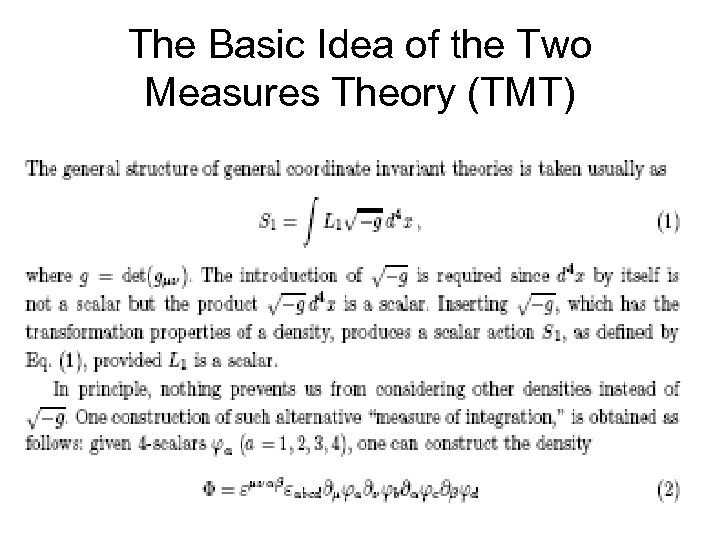The Basic Idea of the Two Measures Theory (TMT)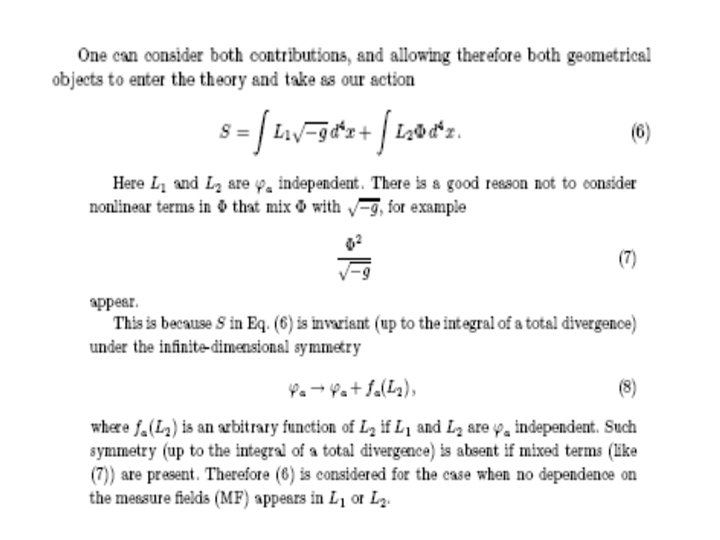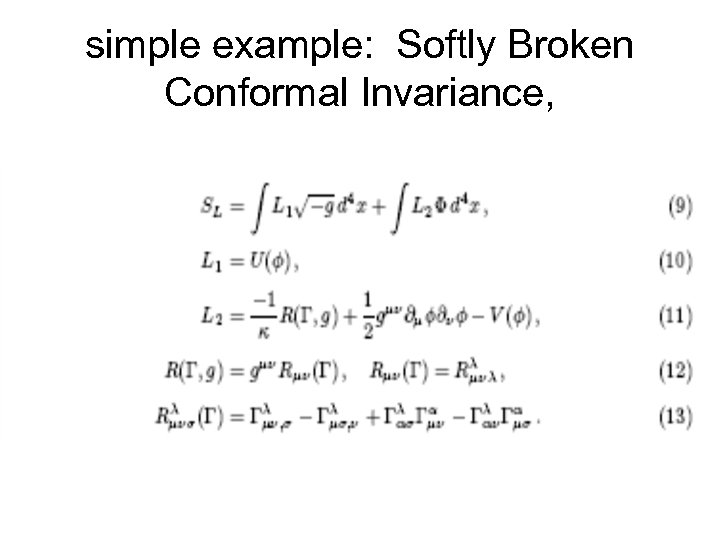simple example: Softly Broken Conformal Invariance,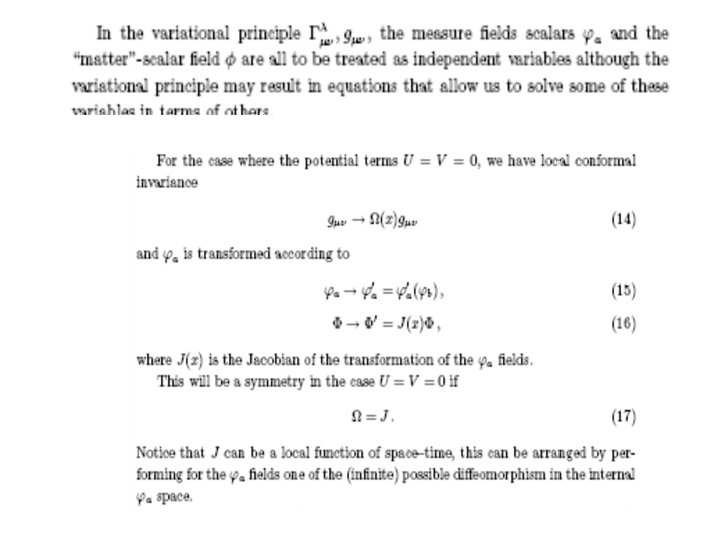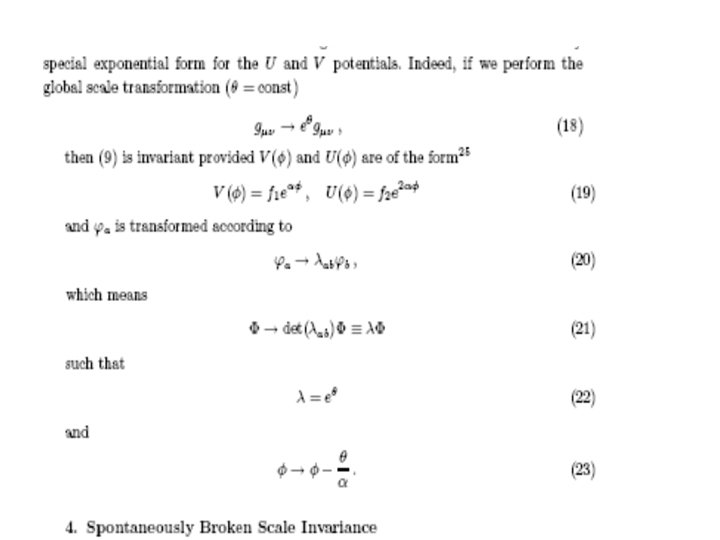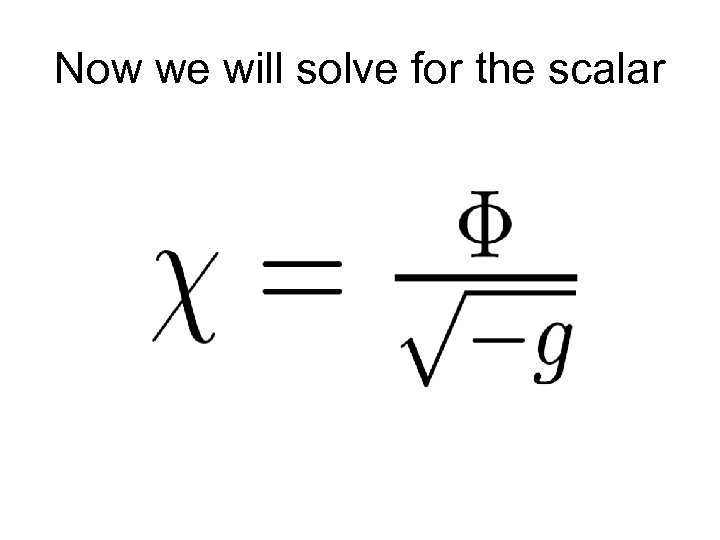Now we will solve for the scalar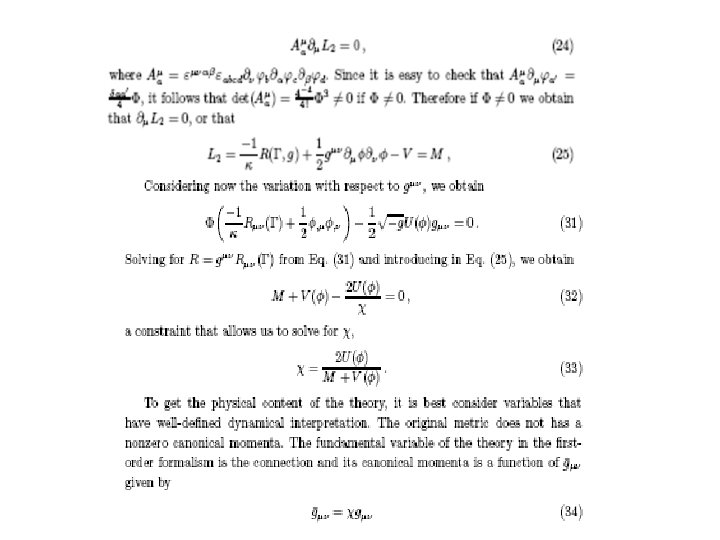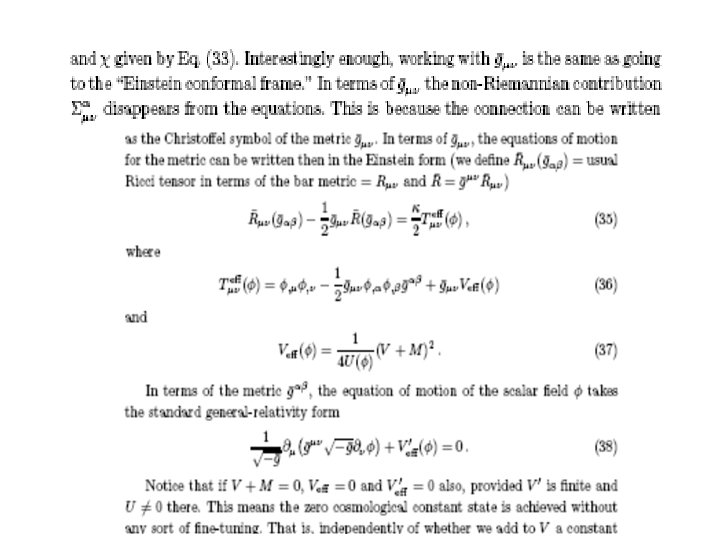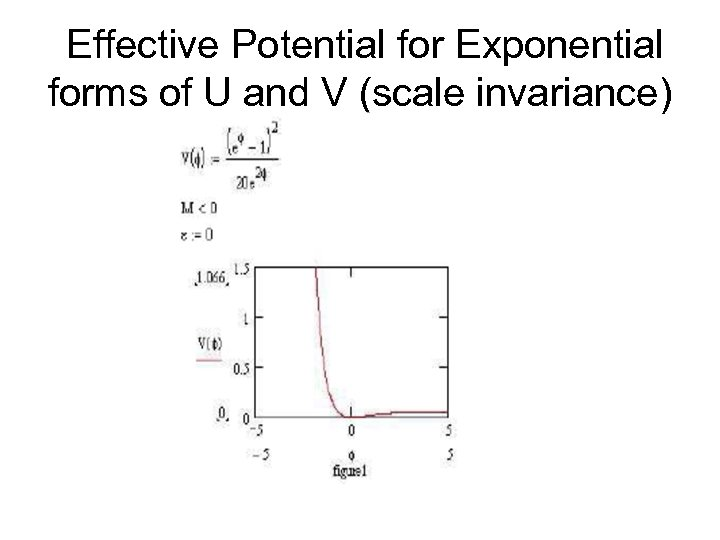Effective Potential for Exponential forms of U and V (scale invariance)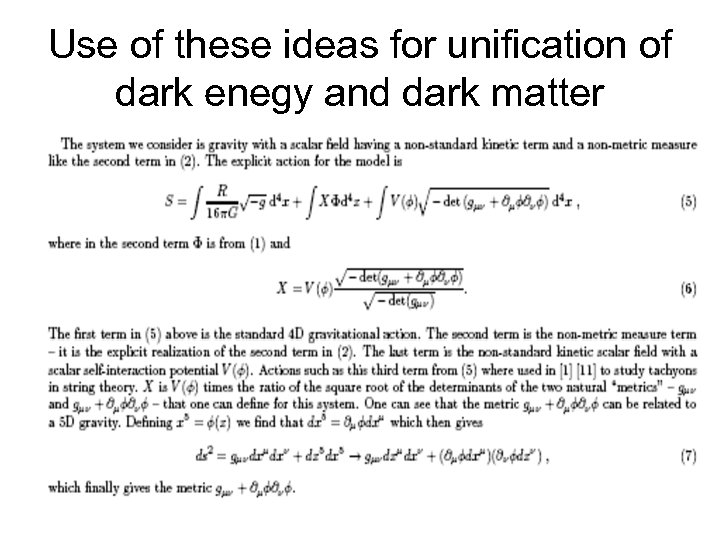Use of these ideas for unification of dark enegy and dark matter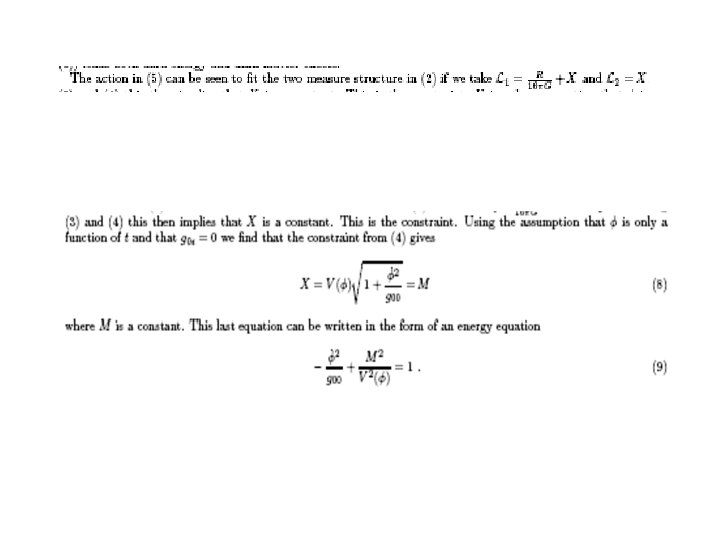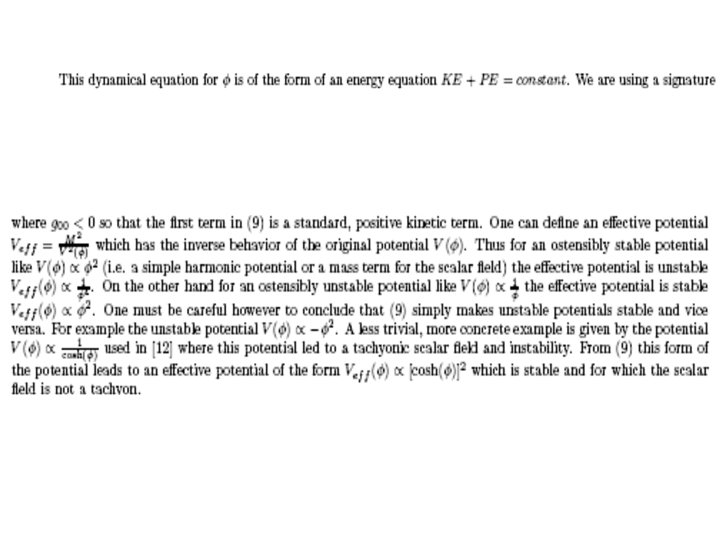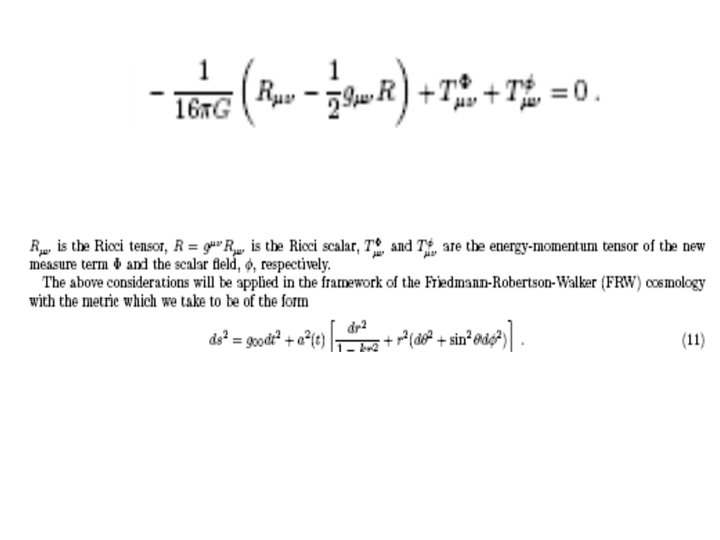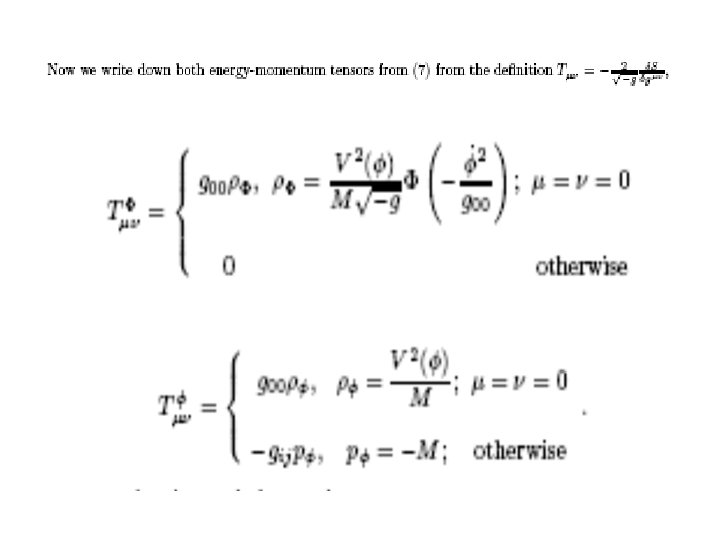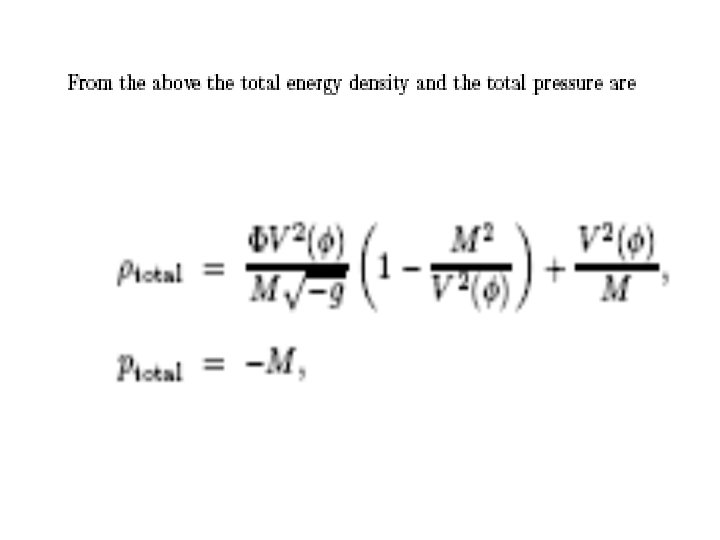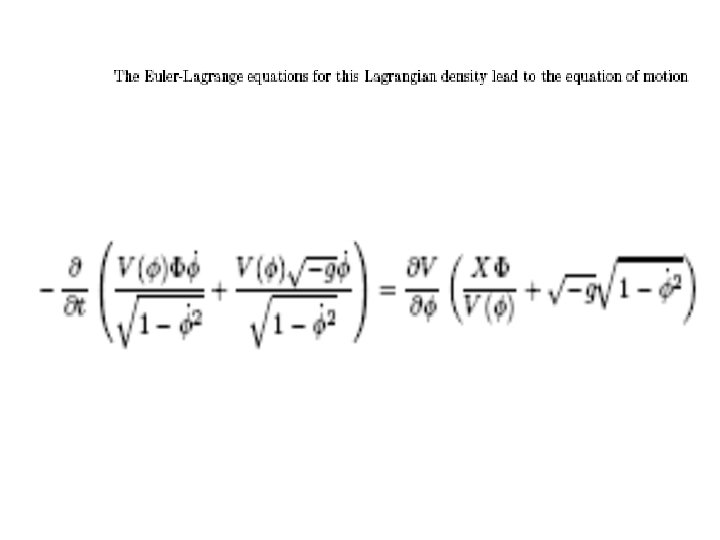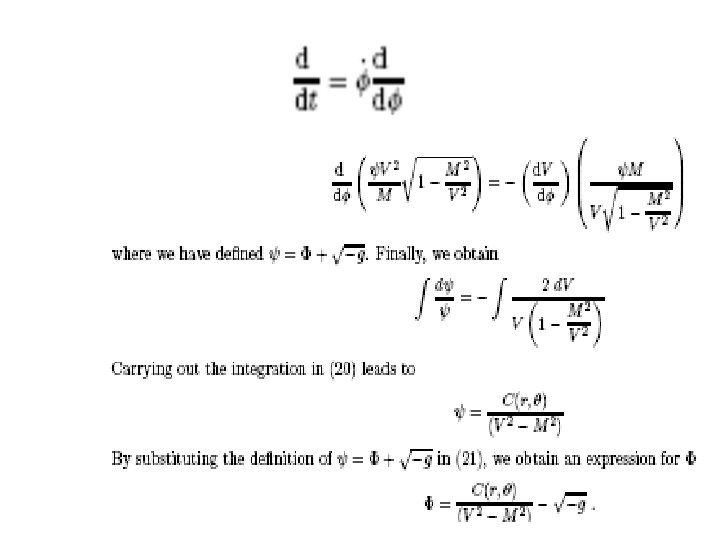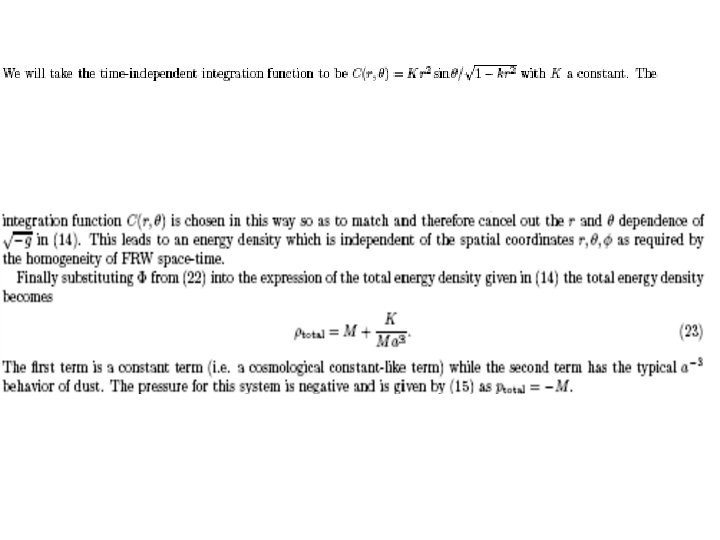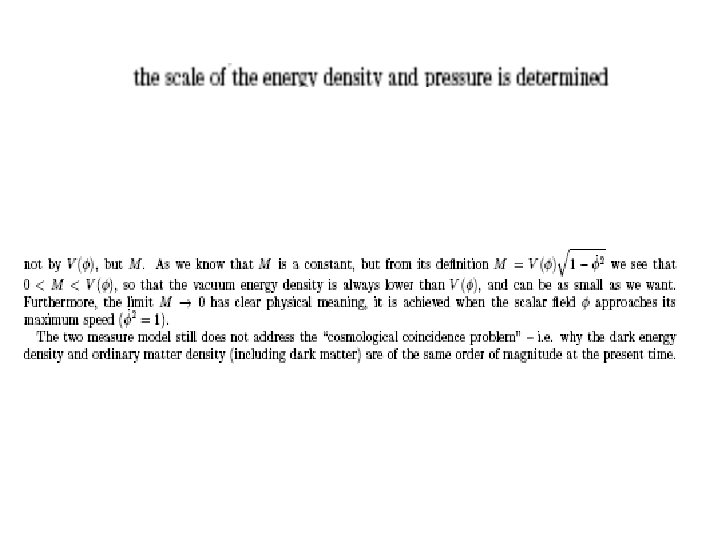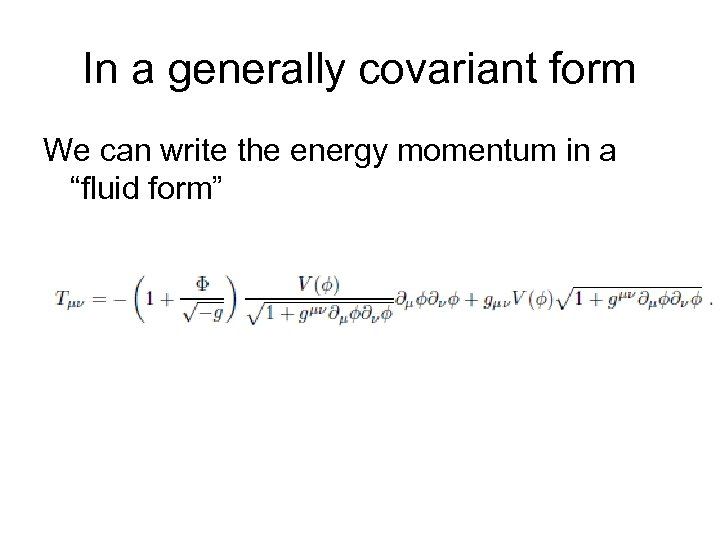In a generally covariant form We can write the energy momentum in a “fluid form”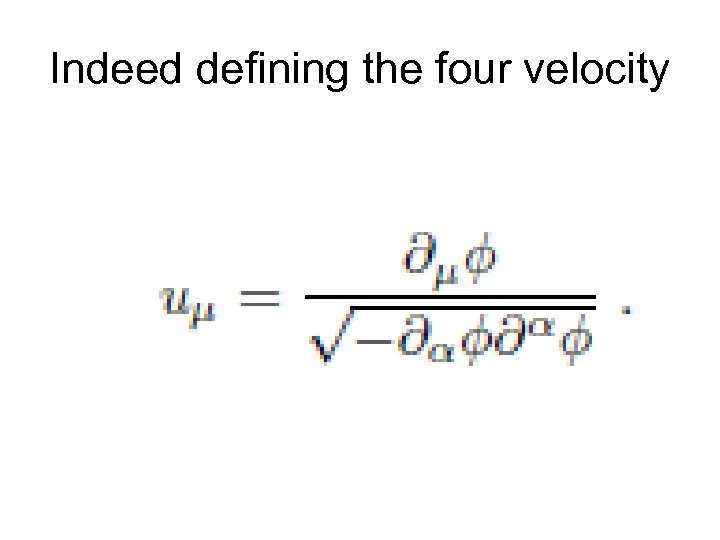Indeed defining the four velocity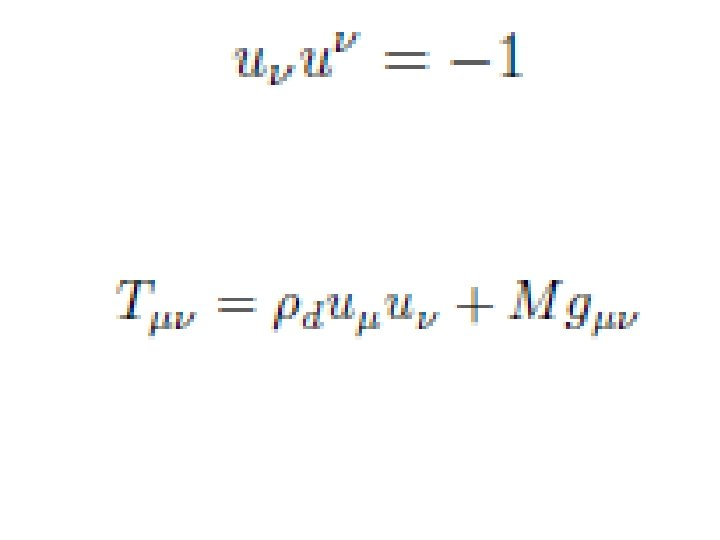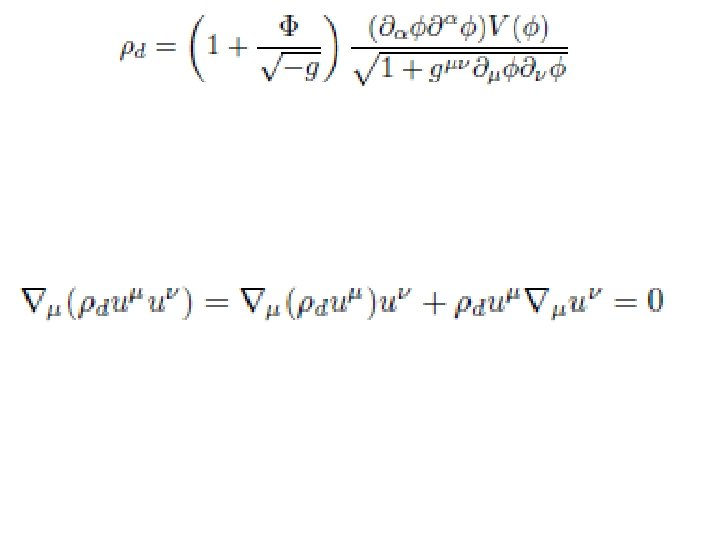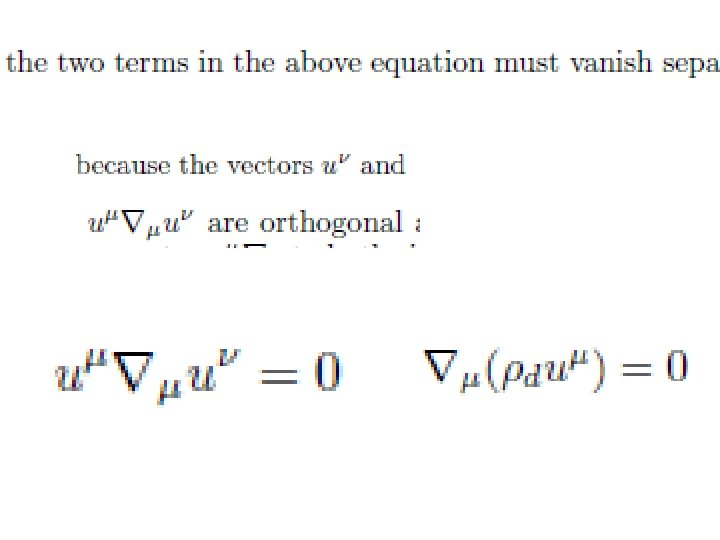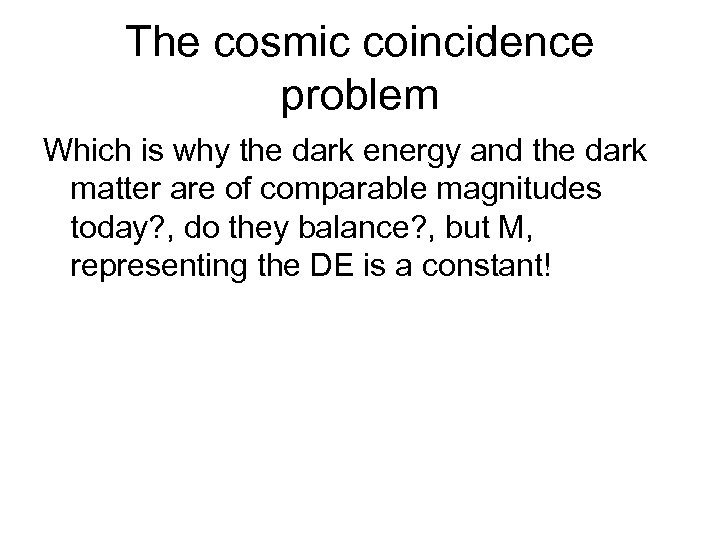The cosmic coincidence problem Which is why the dark energy and the dark matter are of comparable magnitudes today? , do they balance? , but M, representing the DE is a constant!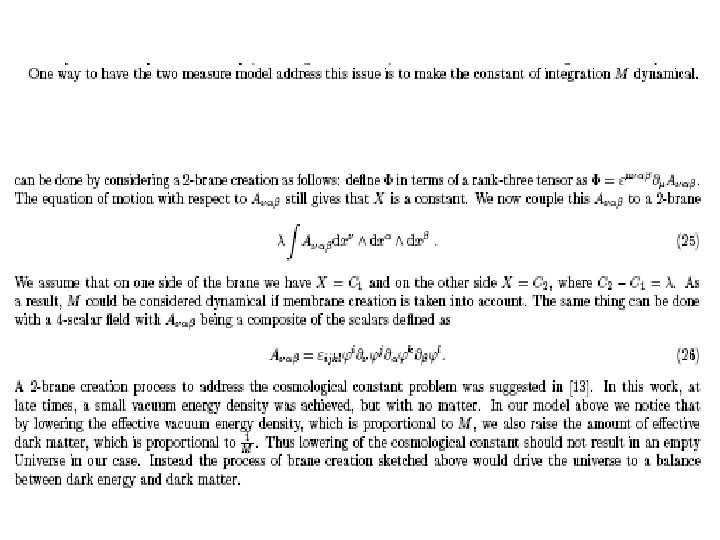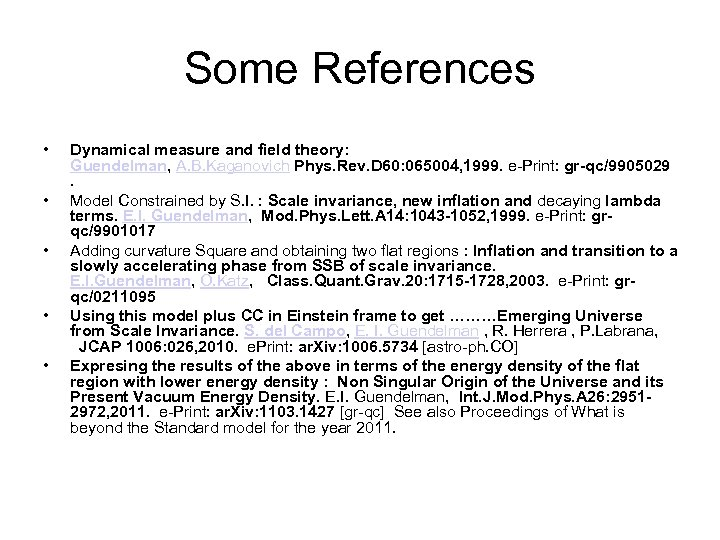Some References • • • Dynamical measure and field theory: Guendelman, A. B. Kaganovich Phys. Rev. D 60: 065004, 1999. e-Print: gr-qc/9905029 . Model Constrained by S. I. : Scale invariance, new inflation and decaying lambda terms. E. I. Guendelman, Mod. Phys. Lett. A 14: 1043 -1052, 1999. e-Print: grqc/9901017 Adding curvature Square and obtaining two flat regions : Inflation and transition to a slowly accelerating phase from SSB of scale invariance. E. I. Guendelman, O. Katz, Class. Quant. Grav. 20: 1715 -1728, 2003. e-Print: grqc/0211095 Using this model plus CC in Einstein frame to get ………Emerging Universe from Scale Invariance. S. del Campo, E. I. Guendelman , R. Herrera , P. Labrana, JCAP 1006: 026, 2010. e. Print: ar. Xiv: 1006. 5734 [astro-ph. CO] Expresing the results of the above in terms of the energy density of the flat region with lower energy density : Non Singular Origin of the Universe and its Present Vacuum Energy Density. E. I. Guendelman, Int. J. Mod. Phys. A 26: 29512972, 2011. e-Print: ar. Xiv: 1103. 1427 [gr-qc] See also Proceedings of What is beyond the Standard model for the year 2011.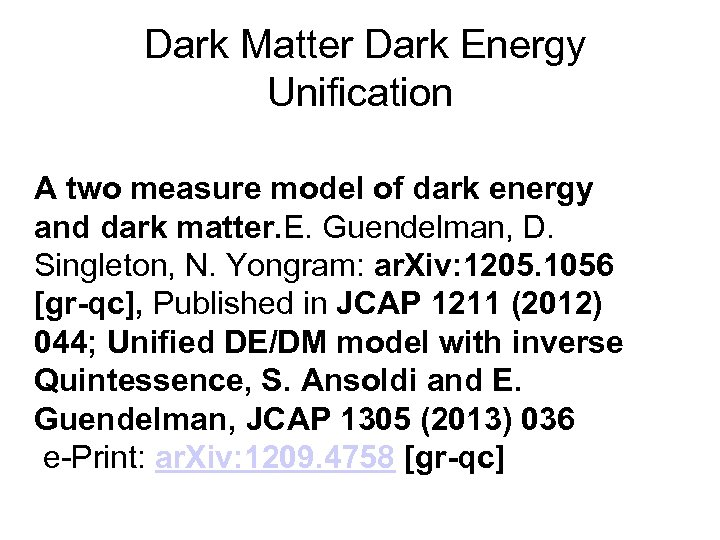Dark Matter Dark Energy Unification A two measure model of dark energy and dark matter. E. Guendelman, D. Singleton, N. Yongram: ar. Xiv: 1205. 1056 [gr-qc], Published in JCAP 1211 (2012) 044; Unified DE/DM model with inverse Quintessence, S. Ansoldi and E. Guendelman, JCAP 1305 (2013) 036 e-Print: ar. Xiv: 1209. 4758 [gr-qc]